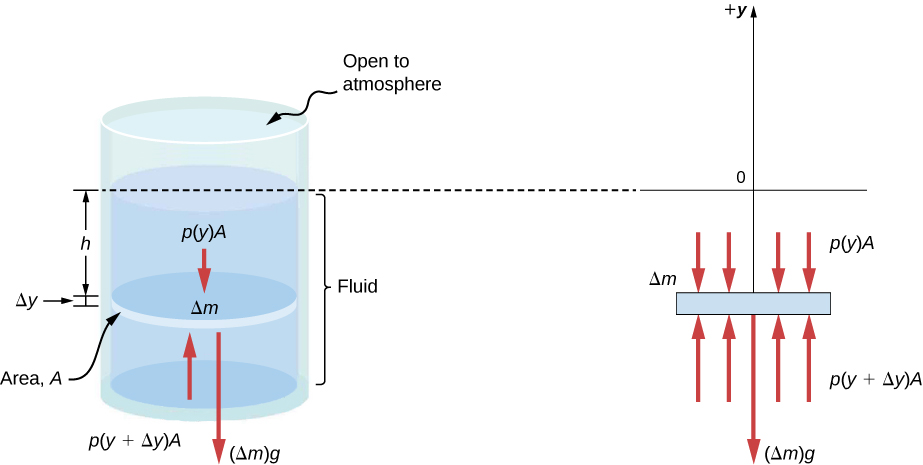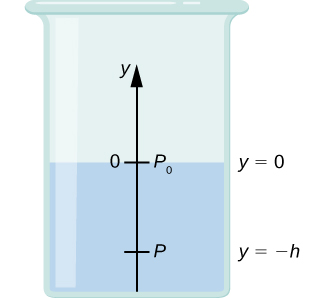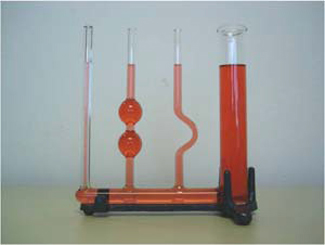14.1 Fluids, density, and pressure  (Page 6/13)

 Page 6 / 13

Imagine a thin element of fluid at a depth h , as shown in [link] . Let the element have a cross-sectional area A and height $\text{Δ}y$ . The forces acting upon the element are due to the pressures p ( y ) above and $p\left(y+\text{Δ}y\right)$ below it. The weight of the element itself is also shown in the free-body diagram.Forces on a mass element inside a fluid. The weight of the element itself is shown in the free-body diagram.

Since the element of fluid between y and $y+\text{Δ}y$ is not accelerating, the forces are balanced. Using a Cartesian y -axis oriented up, we find the following equation for the y -component:

$p\left(y+\text{Δ}y\right)A-p\left(y\right)A-g\text{Δ}m=0\left(\text{Δ}y>0\right).$

Note that if the element had a non-zero y -component of acceleration, the right-hand side would not be zero but would instead be the mass times the y -acceleration. The mass of the element can be written in terms of the density of the fluid and the volume of the elements:

$\text{Δ}m=|\rho A\text{Δ}y|=\text{−}\rho A\text{Δ}y\text{ }\left(\text{Δ}y>0\right).$

Putting this expression for $\text{Δ}m$ into [link] and then dividing both sides by $A\text{Δ}y$ , we find

$\frac{p\left(y+\text{Δ}y\right)-p\left(y\right)}{\text{Δ}y}=\text{−}\rho g.$

Taking the limit of the infinitesimally thin element $\text{Δ}y\to 0$ , we obtain the following differential equation, which gives the variation of pressure in a fluid:

$\frac{dp}{dy}=\text{−}\rho g.$

This equation tells us that the rate of change of pressure in a fluid is proportional to the density of the fluid. The solution of this equation depends upon whether the density ρ is constant or changes with depth; that is, the function ρ ( y ).

If the range of the depth being analyzed is not too great, we can assume the density to be constant. But if the range of depth is large enough for the density to vary appreciably, such as in the case of the atmosphere, there is significant change in density with depth. In that case, we cannot use the approximation of a constant density.

Pressure in a fluid with a constant density

Let’s use [link] to work out a formula for the pressure at a depth h from the surface in a tank of a liquid such as water, where the density of the liquid can be taken to be constant.We need to integrate [link] from $y=0,$ where the pressure is atmospheric pressure $\left({p}_{0}\right),$ to $y=\text{−}h,$ the y -coordinate of the depth:

$\begin{array}{ccc}\hfill {\int }_{{p}_{0}}^{p}dp& =\hfill & \text{−}{\int }_{0}^{\text{−}h}\rho gdy\hfill \\ \hfill p-{p}_{0}& =\hfill & \rho gh\hfill \\ \hfill p& =\hfill & {p}_{0}+\rho gh.\hfill \end{array}$

Hence, pressure at a depth of fluid on the surface of Earth is equal to the atmospheric pressure plus ρgh if the density of the fluid is constant over the height, as we found previously.

Note that the pressure in a fluid depends only on the depth from the surface and not on the shape of the container. Thus, in a container where a fluid can freely move in various parts, the liquid stays at the same level in every part, regardless of the shape, as shown in [link] .If a fluid can flow freely between parts of a container, it rises to the same height in each part. In the container pictured, the pressure at the bottom of each column is the same; if it were not the same, the fluid would flow until the pressures became equal.

Variation of atmospheric pressure with height

The change in atmospheric pressure with height is of particular interest. Assuming the temperature of air to be constant, and that the ideal gas law of thermodynamics describes the atmosphere to a good approximation, we can find the variation of atmospheric pressure with height, when the temperature is constant. (We discuss the ideal gas law in a later chapter, but we assume you have some familiarity with it from high school and chemistry.) Let p ( y ) be the atmospheric pressure at height y . The density $\rho$ at y , the temperature T in the Kelvin scale (K), and the mass m of a molecule of air are related to the absolute pressure by the ideal gas law, in the form

using dimensional analysis find the unit of gravitation constant G in F=G m1 m2/r
Newton meter per kg square
Irtza
meter squre par second and kg swaure
Irtza
what are the possible sources of error in coefficient of static and dynamic friction and there precautions
GRACE
what is Bohr
He is a physicist who formulated the atomic model of an Atom
Lily
And made 3 postulates
Lily
Check university physics vol 3 > Nuclear physics
Lily
Bohr model
kami
what is mean by Doppler effect
Good
increase or decrease in the frequency of sound and light.
Jhon
good
Eng
is it?
Vinayaka
actually it is apparent change in the frequency of light or sound as object move towards or away.
Vinayaka
state the basic assumption of kinetic theory of gases
state the characteristics of gases that differentiate them from solids
FELIX
identify the magnitude and direction a vector quantity
Identify work done on an inclined plane given at angle to the horizontal
DOLLY
formula for Velocity
what is the value of x 6yx7y
what is the formula for frictional force
I believe, correct me if I am wrong, but Ffr=Fn*mu
Grant
frictional force ,mathematically Fforce (Ffr) =K∆R where by K stands for coefficient of friction ,R stands for normal force/reaction NB: R = mass of a body ( m) x Acc.due gravity (g) The formula will hold the meaning if and only if the body is relatively moving with zero angle (∅ = 0°C)
Boay
What is concept associated with linear motion
what causes friction?
Elijah
uneven surfaces cause friction Elijah
Shii
rough surfacea
Grant
what will happen to vapor pressure when you add solute to a solution?
how is freezing point depression different from boiling point elevation?
shane
how is the osmotic pressure affect the blood serum?
shane
what is the example of colligative properties that seen in everyday living?
shane
What is motion
moving place to place
change position with respect to surrounding
to which
to where ?
the phenomenon of an object to changes its position with respect to the reference point with passage of time then it is called as motion
Shubham
it's just a change in position
festus
reference point -it is a fixed point respect to which can say that a object is at rest or motion
Shubham
yes
Shubham
A change in position
Lily
change in position depending on time
bassey
Is there any calculation for line integral in scalar feild?
what is thrust
when an object is immersed in liquid, it experiences an upward force which is called as upthrust.
Phanindra
@Phanindra Thapa No, that is buoyancy that you're talking about...
Shii
thrust is simply a push
Shii
it is a force that is exerted by liquid.
Phanindra
what is the difference between upthrust and buoyancy?
misbah
The force exerted by a liquid is called buoyancy. not thrust. there are many different types of thrust and I think you should Google it instead of asking here.
Sharath
hey Kumar, don't discourage somebody like that. I think this conversation is all about discussion...remember that the more we discuss the more we know...
festus
thrust is an upward force acting on an object immersed in a liquid.
festus
uptrust and buoyancy are the same
akanbi
the question isn't asking about up thrust. he simply asked what is thrust
Shii
a Thrust is simply a push
Shii
the perpendicular force applied on the body
Shubham
thrust is a force of depression while
bassey
what is friction?
MFON
while upthrust is a force that act on a body when it is fully or partially submerged in a liquid
bassey
mathematically upthrust (u) = Real weight (wr) - Apparent weight (wa) u = wr- wa.
Boay
friction is a force which opposes relative motion.
Boay
how did astromers neasure the mass of earth and sun
wats the simplest and shortest formula to calc. for order of magnitude
papillas
Distinguish between steamline and turbulent flow with at least one example of eachByBy Abishek Devaraj